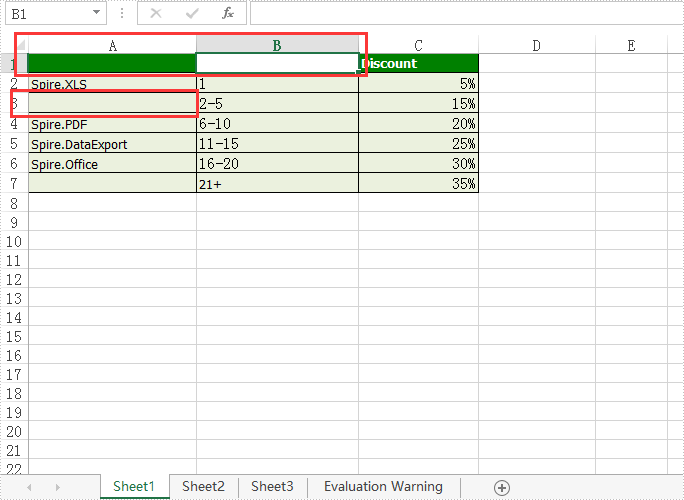Tuesday, 20 June 2017 08:39

## How to remove the value and format from Excel Cell range

Written by  support iceblue
Rate this item

When we operate the data on an Excel worksheet, we may need to delete text from a cell in a spreadsheet document and sometimes even remove all the contents with format from the cell range. This article will demonstrate how to remove the value and format from Excel Cell range with the help of Spire.XLS.

Step 1: Create an instance of Excel workbook and load the document from file.

```Workbook workbook = new Workbook();
```

Step 2: Get the first worksheet from the workbook.

```Worksheet sheet = workbook.Worksheets;
```

Step 3: Set the value as null to remove the original content from the Excel Cell.

```sheet.Range["A1"].Value = "";
```

Step 4: Clear the contents to remove the original content from the Excel Cell.

```sheet.Range["A3"].ClearContents();
```

Step 5: Remove the contents with format from the Excel cell.

```sheet.Range["B1"].ClearAll();
```

Step 6: Save document to file.

```workbook.SaveToFile("result.xlsx",FileFormat.Version2010);
```

Effective screenshot of remove the value and format from Excel Cell range:Full codes:

```static void Main(string[] args)
{

Workbook workbook = new Workbook();

Worksheet sheet = workbook.Worksheets;

sheet.Range["A1"].Value = "";

sheet.Range["A3"].ClearContents();

sheet.Range["B1"].ClearAll();

workbook.SaveToFile("result.xlsx",FileFormat.Version2010);
}
```